# Some Math Tricks I Discovered

Not only will you find tricks in this section, but also some tips to help you with math, & various information about numbers, logic, & geometry. Look out for my characters here, too; they'll make this section more entertaining & educating!

Here's a bonus cartoon for visiting this section: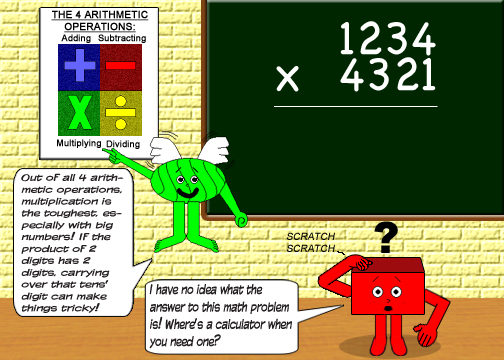If you can multiply big numbers with several digits quickly, then you're a super-genius! By the way, the correct answer to the math problem in the cartoon above is 5,332,114.

## Please select a trick to view:

### Note: If I discover any more math tricks, then I'll add them to this list!

Here's a bonus fact: -40 degrees Farenheit is equal to -40 degrees Celsius!

Proof: The 2 conversion functions intersect at x = -40, y = -40

F = 9/5 × C + 32

C = 5/9(F - 32)Another bonus fact! This is an interesting fact about the number 2, but you probably already knew it!Another bonus fact!The reciprocal of a number's square root is equal to the square root divided by the original number! (x is not equal to zero!)

Here's a fact about checkerboards! (Or you can also call them chessboards) You can calculate how many squares a checkerboard has with the 3 formulas below:

### n2 + (n - 1)2 + (n - 2)2 + ... + 32 + 22 + 12

#### which is the same as:(x is also equal to the number of rows & columns!)

### n3/3 + n2/2 + n/6

The 1st formula is in geometric series form, the 2nd one is in summation form and the 3rd is in cubic function form.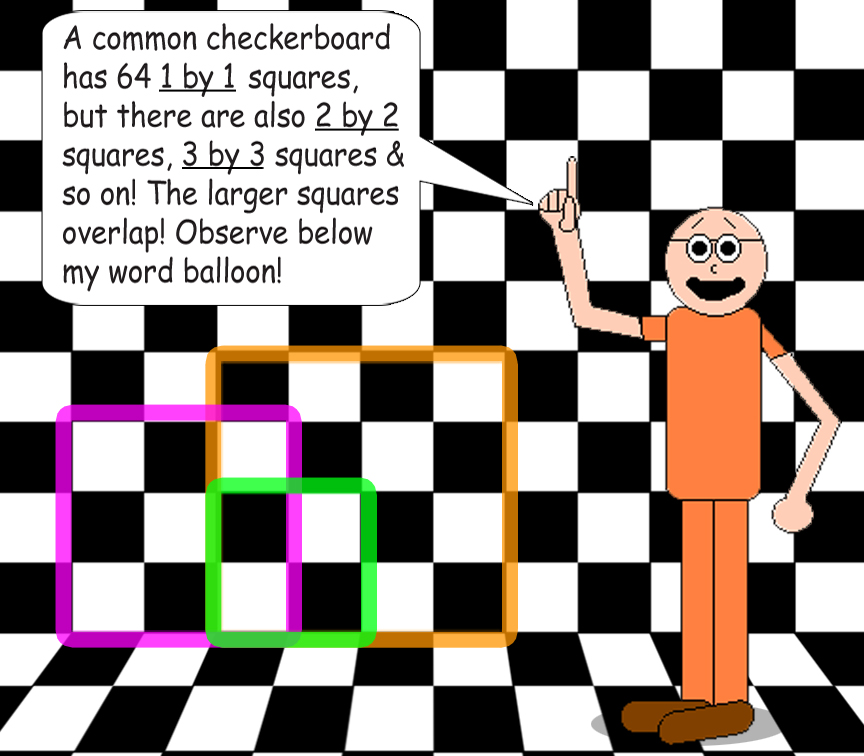Here's a bonus fact about permutations & combinations:

If you pick 2 objects at a time from any set that has at least 2 objects, the permutation-to-combination ratio is always equal to 2. In other words, there will be half as many combinations as permutations.

Here's a bonus fact about the suffix -illion:

What does the suffix mean? Well, here's the -illion function!

Y = 10^(3X + 3)

X = the prefix's number, Y = the actual number

For example, 1 sexagintillion is a 1 followed by 183 zeroes! The prefix sexaginti- means "60"; 3 × 60 + 3 = 183.

Note: The number you pick for the variable x must be a counting number.

Here's the inverse of the -illion function!

X = (log(Y) - 3)/3

P.S.: About dividing by 3 in the inverse of the -illion function:

• If the remainder in the quotient is 1, then Y = ten "X"-illion.

• If the remainder in the quotient is 2, then Y = hundred "X"-illion.

• If there's no remainder in the quotient, then Y = "X"-illion.

For example, the googol is a 1 followed by 100 zeroes, so according to the inverse of the -illion function, it's also 10 dotrigintillion since the prefix dotriginti- means "32" & (log 10100 - 3)/3 = (100 - 3)/3 = 97 ÷ 3 = 32 + 1/3; the remainder is 1 in this case.

Here's a bonus fact about imaginary/complex numbers: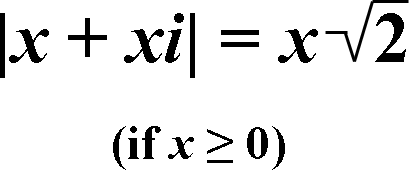P.S.: If x is a negative number, then it's -x times the square root of 2.

Here's a bonus fact about triangles:

### Let A, B & C be the 3 angles of a triangle. Then...

sin(A + B) = sin(C)

sin(B + C) = sin(A)

sin(C + A) = sin(B)

In other words, the sine of the sum of 2 angles in a triangle will always be equal to the sine of the 3rd remaining angle!

Here's a bonus fact about birth years & age, measured in full years of course:

### If the number of your birth year is odd, then your age will be even in odd-numbered years & odd in even-numbered years!

Another bonus fact!

### After eating a power pellet...

 If Pac-Man eats 1 ghost: If Pac-Man eats 2 ghosts: If Pac-Man eats 3 ghosts: If Pac-Man eats all 4 ghosts: 1st Ghost 200 200 200 200 2nd Ghost 400 400 400 3rd Ghost 800 800 4th Ghost 1600 Sums: 200 600 1400 3000

(This is referring to the original version of the video game)

The number of points earned for each ghost refers to this summation formula:See? Even video games have math in them!

Here's a bonus fact about the Law of the Cosines: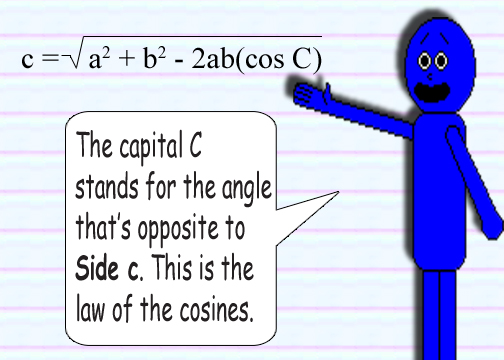If Angle C = 90°, then the formula simplifies to the original Pythagorean theorem (since the cosine of a right angle is zero); but if Angle C = 180°, then the formula simplifies to:

c = a + b

...Because cos(180°) = -1. (That's right! Side c simply becomes the sum of Sides a & b! However, the figure would be a straight line instead of a triangle!)

Here's a bonus fact that I call my Prime Number Test:

### If an integer is not divisible by any prime numbers that are less than its square root, then it is also a prime number!

Here's a bonus fact about fractions:According to this cartoon, if the absolute value of the difference between the numerator & denominator is 1, then the fraction is already in its simplest terms! By the way, these 2 functions never intersect! Also, they're both discontinuous functions because of division by zero!

Here's a bonus fact about logarithms:

logxY ÷ logxZ = logzY; none of the 3 variables are equal to zero! Neither X nor Z equals 1.

Here's a bonus fact about trigonometry:

tan X = (sin X)/(cos X) = (sec X)/(csc X) = 1/(cot X)

### AND

cot X = (cos X)/(sin X) = (csc X)/(sec X) = 1/(tan X)

That's right! These are the classic Tangent & Cotangent Formulae! They both work with secants & cosecants, too! For more details, see the math tricks about trigonometry.

Here's another bonus fact about imaginary/complex numbers:

### A complex number's square always has an angle that's twice the size of the 1st angle!

(If neither a nor b are negative, considering a + bi)

### Example:

(1 + i)2 = 2i; angle(1 + i) = 45°, so angle(2i) = 90° and 45 × 2 = 90

Here's another bonus fact about logarithms:

(logxY)-1 = logYX; neither X nor Y equals 1 or 0.

Yet another bonus fact about logarithms:

log10^X(10Y) = Y/X; X ≠ 0

Note: You can substitute 10 with another number & get the same fraction! (But don't pick 0 or 1)

Here's another bonus fact:

### Because:

(3i)2 ≠ 3i2; (3i)2 = -9 & 3i2 = -3

Here's a bonus fact about right isosceles triangles: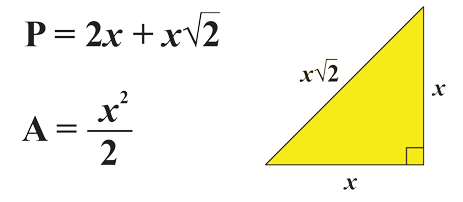P stands for the perimeter, A stands for the area & x = the length of 1 of the 2 equal sides(also called legs).

Note: The perimeter-to-area ratio will be 1:1 if x = 4 + 2 square roots of 2; the perimeter will be 12 + 8 square roots of 2 units & the area will be 12 + 8 square roots of 2 units2!

Here's a bonus fact about the function y = x2:What those math symbols in T.V. Man's screen are saying is that for the function y = x2, if x is equal to a fraction that has a power of 2 as the denominator, then the numeric derivative is always equal to n/(2m-1)!

n = the numerator of your choice, m = the power of 2 of your choice, x = n/2m

Here's a bonus fact about integrals:

If B > A, thenThe variable C is equal to any real number you want it to be!

Here's a bonus fact about dividing fractions:

A/B ÷ C/D = (C/D ÷ A/B)-1; none of the variables are equal to zero(0)

### Example:

5/3 ÷ 6/7 = 35/18 and 6/7 ÷ 5/3 = 18/35

Notice how the quotients of the division problems are reciprocals of each other! Also, by the way...

5/3 = 1 + 2/3 and 35/18 = 1 + 17/18

(The numerator isn't always less than the denominator!)

You know that raising a number to the power of -1 gives you the reciprocal, don't you? Whenever negative numbers are exponents, numerators become denominators & vice versa!

By the way, fractions can be printed horizontally or vertically. The numerator is the number on top/to the left of the vinculum & the denominator is the number on the bottom/to the right of the vinculum.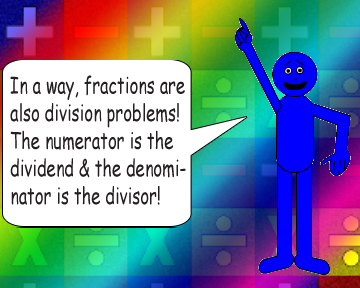In other words, remember:

X ÷ Y = X/Y

Another thing to remember about division:Here's another bonus fact about imaginary/complex numbers:

### Example:

|1 + i| = √(2); angle(1 + i) = 45°

|2 + i| = √(5); angle(2 + i) ≈ 26.565°

(1 + i)(2 + i) = 1 + 3i; angle(1 + 3i) ≈ 71.565°; |1 + 3i| = √(10)

45° + 26.565° = 71.565° and √(2) • √(5) = √(10)

(1 + i) ÷ (2 + i) = 3/5 + 1/5i; angle(3/5 + 1/5i) ≈ 18.435°; |3/5 + 1/5i| = √(2/5)

45° - 26.565° = 18.435° and √(2) ÷ √(5) = √(2/5)

Here's another bonus fact about division & the powers of denominators of fractions:

Note: Watch the parentheses carefully!

(n/(dx+1)) ÷ (n/(dx)) = 1/d; neither d nor n are equal to zero(0)!

Here's a bonus fact about exponentiation with unit fractions:Here's a bonus fact about multplying reciprocals:

A-1B = (AB-1)-1 & AB-1 = (A-1B)-1; neither A nor B are equal to zero(0)!

Here's a bonus fact about mixed numbers: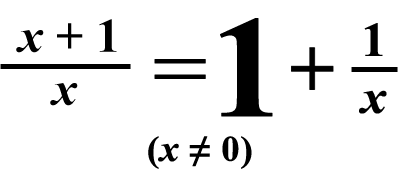Note: Even if the variable x is not an integer, the 2 functions coincide!

If x = φ or -φ + 1, then so is y!

Here's a bonus fact to remember about negative numbers vs. positive numbers:

If X > Y, then -X < -Y

Here's a pair of bonus facts about trigonometry:

• The sine & secant functions don't intersect.
• The cosine & cosecant functions don't intersect.

Here's a fact about the formula for the area of a circle:

D • (πR)/2 = (πD2)/4 = πR2

You can multiply the diameter by a quarter of the circumference to get the area! Also, Pi(π) times the diameter squared, divided by 4 equals Pi(π) times the radius squared!

Here's another fact about the formula for the area of a circle:

You can differentiate it to the formula for the circumference!Here's a fact about the formula for the volume of a sphere:

You can differentiate it to the formula for the surface area!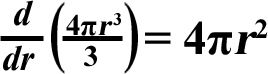(This is like the 3-dimensional version of the fact just above this one!)

Speaking of numbers, here are some Web pages with interesting facts about specific numbers: